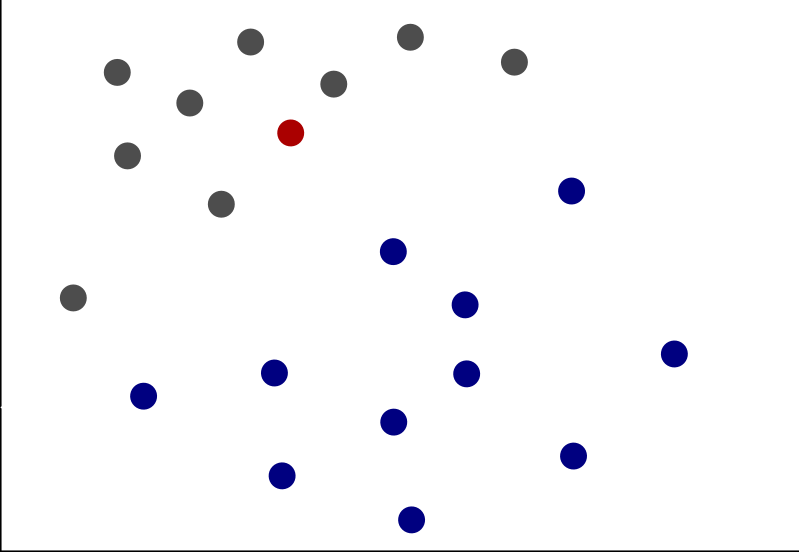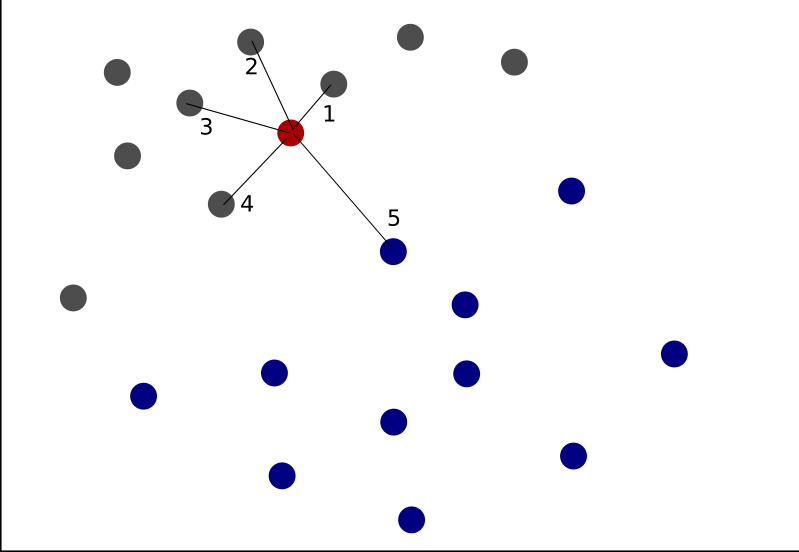## Basic Supervised Learning Overview

There are some really good tools for supervised learning out there. Every popular language has them. Most of the existing toolkits will have all of the algorithms mentioned here plus a lot more. If you’re comfortable with Python, I suggest starting with scikit learn since it has a standard interface for all of its machine learning techniques.

### Gestalt

Machine learning is about making predictions based on pattern recognition. As an example, suppose we wanted to predict whether the dot marked in red belongs with the blue group or the grey group.There are many ways to make a prediction as to the appropriate classification for this dot, but perhaps one of the simplest machine learning techniques is the k-Nearest Neighbors algorithm. K-Nearest Neighbors is prediction based on the weighted values of other observations with predictive attributes that are close to that of the item in question. The k can represent any number of observation which can be averaged together to make the final prediction. For this example, let’s use a k value of 5.For the point we’re trying to identify, 4 / 5 of the nearest neighbors belong to the grey classification, so it is very likely that the correct classification for this point should also be grey. In order to be confident in the predictive power of our model, however, we would first want to test our prediction accuracy on data that we already know the classification for.

### Setup

Whichever algorithm you choose, the setup will be pretty much the same. First, you’re going to need some data that have already been classified so that whichever algorithm you choose will have something to learn from. Next, you’ll want to split the classified data into two sections : a training set and a test set. The training data will allow the algorithm to generate hypothetical models to fit them and the test data will enable the algorithm to avoid overfitting. Finally, since the the data in your training and test sets are already classified, the accuracy for how the model performs on the classified data should give a fairly reasonable expectation of what the accuracy will be for the unclassified data.

### Example

Naive Bayes

Support Vector Machines

Decision Tree Learning